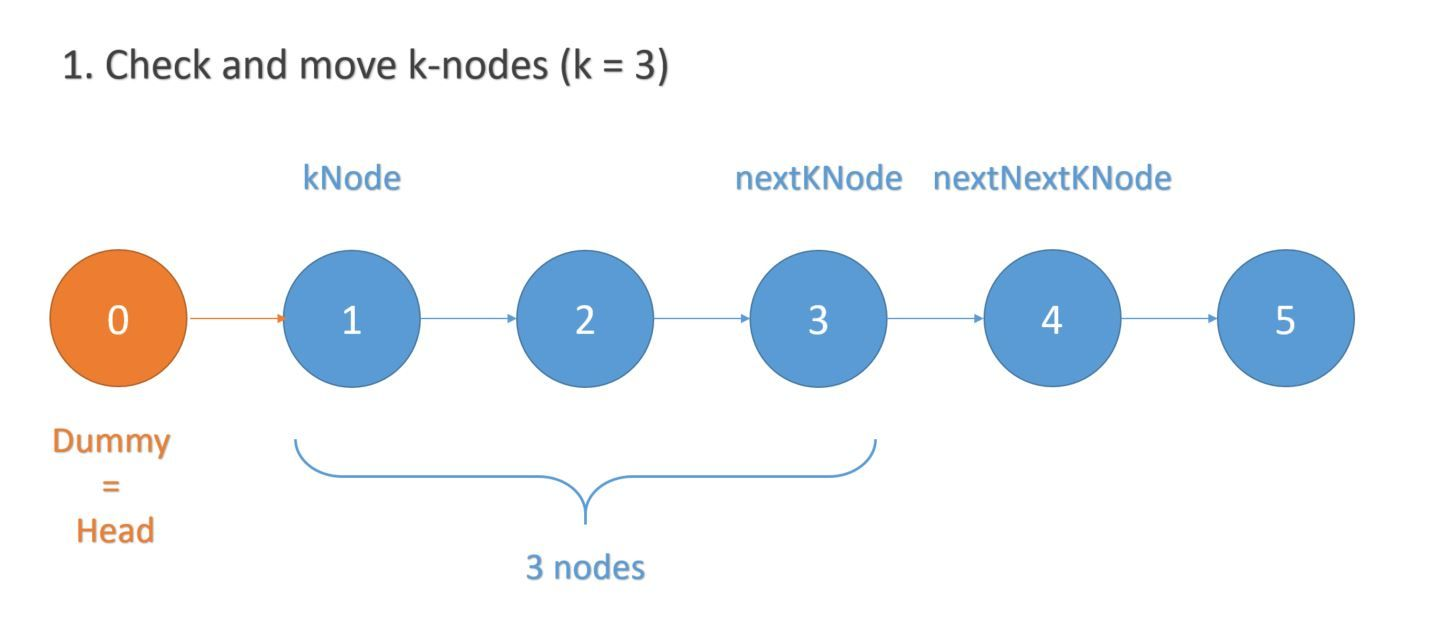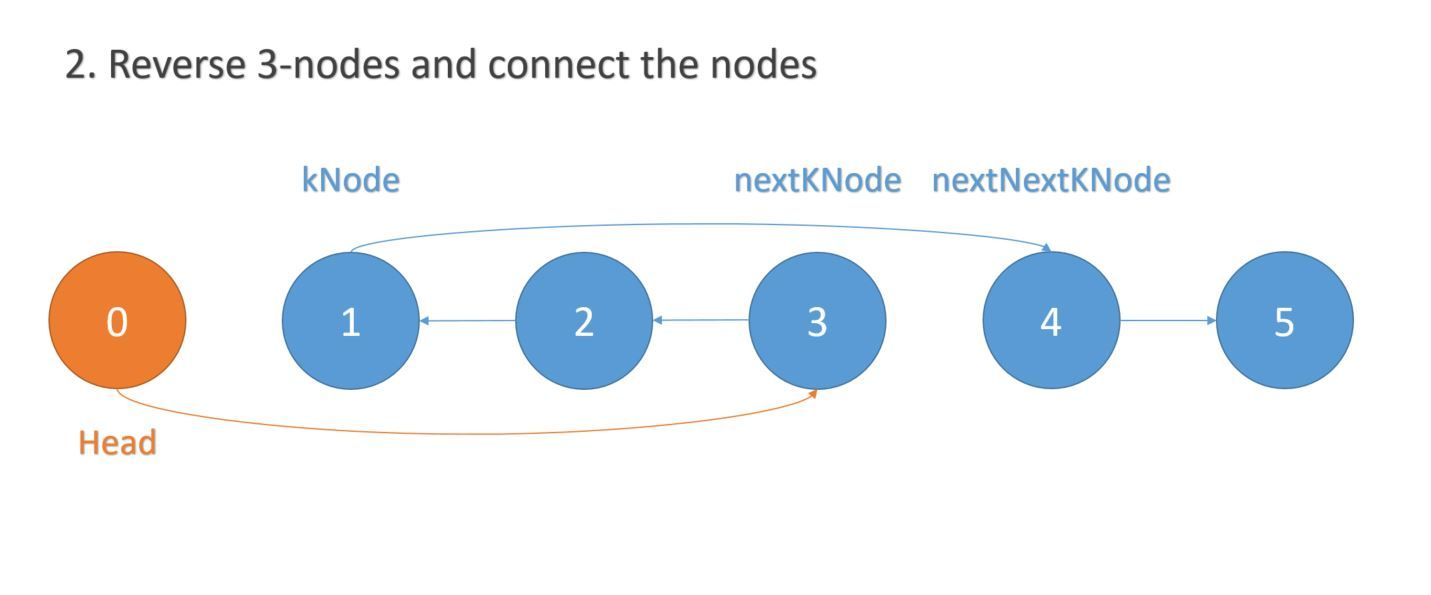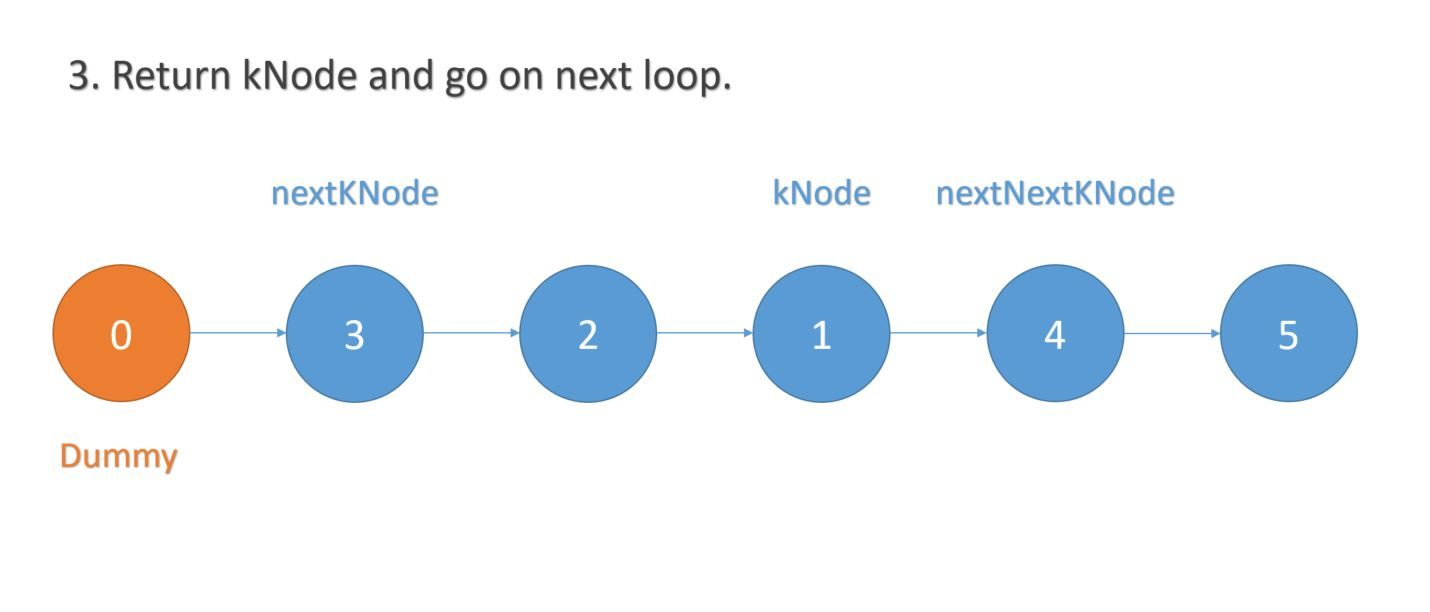### Given a Linked List, reverse the nodes of a linked list `k` at a time and return its modified list.

The `k` value is a positive integer and less than or equal to the length of linked list. If the number of nodes is not a multiple of `k` (less than `k`), then we do not need to reverse those remaining nodes.

For example,

``````Given k = 2 and list 1->2->3->4->5, reorder it to 2->1->4->3->5.

Given k = 3 and list 1->2->3->4->5, reorder it to 3->2->1->4->5.
``````

Let's look at the solution together:

``````    public ListNode reverseKGroup(ListNode head, int k) {

if(head == null || k == 0){
}

ListNode dummy = new ListNode(0);

while( true ){
// get the head of reversed nodes (node before actual reverse)
break;
}
}

return dummy.next;
}

// reverse the k-nodes
private ListNode reverseKNodes(ListNode head, int k){

// get the k-th node
for (int i = 0; i < k; i++) {
if (nextKNode == null || nextKNode.next == null) {
return null;
}
nextKNode = nextKNode.next;
}

ListNode nextNextKNode = nextKNode.next;

ListNode prev = null;
ListNode curr = kNode;

// reverse k-nodes
for(int i = 0; i < k ; i++){
ListNode temp = curr.next;
curr.next = prev;
prev = curr;
curr = temp;
}

// connect the head and tail of reversed list
kNode.next = nextNextKNode;

return kNode;
}
``````

The steps are very straightforward:

1. check if the k-nodes is reversible
2. reverse k-nodes and keep looping until k-nodes is not reversible.

Here's some visual graph to assist you understanding the code:That's all! Hope you enjoy it!

Post was published on , last updated on .

Like the content? Support the author by paypal.me!